# Problem 58977. Find the smallest number leading to a maximal product of two numbers that concatenate to another number

Cody Problem 58971 involves the maximal product of numbers that concatenate to a number n. For example, if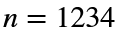, then the products are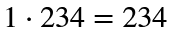,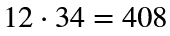, and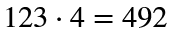, and the maximal product is 492. For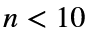, the maximal product is zero.
This problem seeks the smallest number leading to a given maximal product. If the product is 492, the smallest number is 682. Some products have no solution. For example, 13 is a product of both 113 and 131, but the maximal products are 33 and 31, respectively.
Write a function that takes a maximal product and returns the smallest number leading to that product. If the product has no corresponding number, return the empty set.

### Solution Stats

50.0% Correct | 50.0% Incorrect
Last Solution submitted on Sep 19, 2023

### Community Treasure Hunt

Find the treasures in MATLAB Central and discover how the community can help you!

Start Hunting!